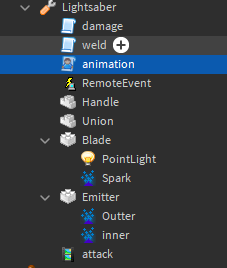# Lightsaber animation not working

So I’m making a light saber for a friend, and had to follow a tutorial for some of the scripting. But for what ever reason my animation is not working.

I also would like to add sound to this but not sure how to do that with out messing up the other code. For the sound I want it to play a sound when I press “j” to turn it on and a different sound when I click on my screen.

Animation: attack 2.0 R6 - Roblox

``````local uis = game:GetService("UserInputService")

local tool = script.Parent
local attack = tool:WaitForChild("attack")

local player = game.Players.LocalPlayer
local equipped = false

local on = false
tool.Activated:Connect(function()
if not on then return end

animation:Play()
end)

tool.Equipped:connect (function	()
equipped = true
end)

tool.Unequipped:connect (function	()
equipped = false
end)

local function turnOff()
on = false
tool.RemoteEvent:FireServer(false)
end

local function turnOn()
on = true
tool.RemoteEvent:FireServer(true)
end

uis.InputBegan:Connect(function (input, processed)
if processed then return end

if input.KeyCode == Enum.KeyCode.J and equipped then
if on then
turnOff()
else
turnOn()
end
end

end)

``````1 Like

You misspelled something here:

``````local animation = player.Character.Humanoid:LoadAninmation(attack)
``````

Take a guessNote: You should add if there are any errors in the script or in the output.

Oh… lol scripting at 10 pm wasn’t a good idea huh.

it depends what sound you want to use. If the sound is for turning on the light saber, put it in the place that receives the remote event as “true”. If the sound is for the light saber attack, put it right before the animation.

(Sorry for taking so long to respond)

I have one that will be the start so when they press “j” to power it on and one for while is idle

If the input thingy works, then all you need to do is input the sound in the following:

``````uis.InputBegan:Connect(function (input, processed)
if processed then return end

if input.KeyCode == Enum.KeyCode.J and equipped then
if on then
turnOff()
--here for when turned off
else
turnOn()
--here for when turned on
end
end

end)
``````

I think you can just put the sound object in the tool, or the player. Here’s what it would look like in the tool:

``````local sound = tool.sound --Note: sound is the example name of the sound. replace it with whatever your sound name is. Also remember to actually add a sound object in the tool and input the correct id.
uis.InputBegan:Connect(function (input, processed)
if processed then return end

if input.KeyCode == Enum.KeyCode.J and equipped then
if on then
turnOff()
sound:Play() --Play the sound when the thing turns off
--here for when turned off
else
turnOn()
--here for when turned on
sound:Play() --Play the sound when the thing turns on
end
end

end)
``````

Here’s the Sound article from DevHub in case you need it.

Question- do you just want to know when to use the sound? or do you need the thing where you press J part as well? looks like you’ve already got it here:

``````uis.InputBegan:Connect(function (input, processed)
if processed then return end

if input.KeyCode == Enum.KeyCode.J and equipped then
if on then
turnOff()
else
turnOn()
end
end

end)`````````

I just edited one of my replies- go look at it and see if it solves your sound problem

should i make a new script of place this in the animation one? if so where?

I have it so when they press j the lightsaber turns on. So I want it to also make a sound when it dose that.

find the similar part in your code and replace it. (aka, this part)

``````uis.InputBegan:Connect(function (input, processed)
if processed then return end

if input.KeyCode == Enum.KeyCode.J and equipped then
if on then
turnOff()
else
turnOn()
end
end

end)
``````

The “press J to turn off/on” works right? if so just do that

I just did that and now its not working

``````local uis = game:GetService("UserInputService")

local tool = script.Parent
local attack = tool:WaitForChild("attack")

local player = game.Players.LocalPlayer
local equipped = false

local on = false
tool.Activated:Connect(function()
if not on then return end

animation:Play()
end)

tool.Equipped:connect (function	()
equipped = true
end)

tool.Unequipped:connect (function	()
equipped = false
end)

local function turnOff()
on = false
tool.RemoteEvent:FireServer(false)
end

local function turnOn()
on = true
tool.RemoteEvent:FireServer(true)
end

local sound = tool.lightsaberOn
uis.InputBegan:Connect(function (input, processed)
if processed then return end

if input.KeyCode == Enum.KeyCode.J and equipped then
if on then
turnOff()
sound:Play() --Play the sound when the thing turns off
--here for when turned off
else
turnOn()
--here for when turned on
sound:Play() --Play the sound when the thing turns on
end
end

end)
``````
1. is the sound supposed to be played when turning off AND on?
2. was the J key pressing detection system working?
3. Does the animation work?
4. Any errors? (output and script analysis)

just when turning on
yes
not anymore
nope.

here’s a clip of hat it looked like before
https://gyazo.com/0c4f4c2bbb9ed520f4e83ed12edc2b04

Sorry I slept-

First, try removing the one with the sound playing when turning off

Does the sound work when turned on, but the animation part doesn’t work?